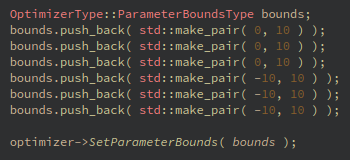# particle swarm optimizer

Hello everyone,
I was wondering if itk developers provided a registration example between two 3d images using particle swarm optimizer ? Thank you

I don’t think there is such an example, but you can be inspired by particle swarm’s unit test and a registration example of your choice (perhaps this one).

ok, thank you @dzenanz

I tried your solution @dzenanz and when I execute my program it stops just before finishing the registration process with this error message: `Description: itk::ERROR: ParticleSwarmOptimizer(0x5578e8497ac0): cost function and parameter bounds dimensions mismatch `
My question is: how can I declare a cost function?

okay, I managed to understand where the error came from. Just another question: what is `OptimizerType::ParameterBoundsType bounds;`?
If I use 3d euler coordinates system, do I have to initialize `bounds` like this:?
Where the first three pushbacks bounds euler angles and the three last pushbacks bounds the translation among x, y, and z?

Use your IDE to explore the exact definition of this type.

You initialization with 6 pairs of values is good in principle, but I don’t know whether it compiles.

again and again, thank you @dzenanz, my program works fine now

Maybe explain what was the exact problem and solution, in case somebody comes across this thread in the future?

excuse me for the late reply. My problem was in fact very basic: I had a segfault when launching my registration program which uses a particle swarm optimizer. I just had to declare `ParameterBoundsType` and `push_back()` some `double` values in it.
The first three `push_back()` concerns Euler rotation bounds, the three last translation bounds.
Here is a sample of code which compiles and runs:

``````const uint16_t MAXIMUM_NUMBER_OF_ITERATIONS = 100;
const uint16_t RANDOM_SEED = 50;
const bool SEED = true;
const double GLOBAL_COEFFICIENT = 1.1;
const double INERTIA_COEFFICIENT = 0.6;
const double PERSONAL_COEFFICIENT = 1.1;
const double NUMBER_OF_GENERATION_WITH_MINIMAL_IMPROVMENT = 10;
const double NUMBER_OF_PARTICLES = 100;
const double PERCENTAGE_PARTICLES_CONVERGED = 15;
const double X_TOLERANCE = 0.01;
const double F_TOLERENCE = 0.01;
const bool INITIALIZE_WITH_NORMAL_DISTRIBUTION = true;
const double EULER_ANGLES_BOUNDS = 0.1; // in degrees
const double XYZ_TRANSLATION_BOUNDS = 0.1; // in mm
const double ROTATION_TRANSLATION_OPTIMIZER_VALUE = 1; // divids rotation and translation parametric space by this value

optimizer->SetGlobalCoefficient( GLOBAL_COEFFICIENT );
optimizer->SetInertiaCoefficient( INERTIA_COEFFICIENT );
optimizer->SetPersonalCoefficient( PERSONAL_COEFFICIENT );
optimizer->SetMaximalNumberOfIterations( MAXIMUM_NUMBER_OF_ITERATIONS );
optimizer->SetNumberOfGenerationsWithMinimalImprovement( NUMBER_OF_GENERATION_WITH_MINIMAL_IMPROVMENT );
optimizer->SetNumberOfParticles( NUMBER_OF_PARTICLES );
optimizer->SetPercentageParticlesConverged( PERCENTAGE_PARTICLES_CONVERGED );
optimizer->SetSeed( RANDOM_SEED );
optimizer->SetUseSeed( SEED );
optimizer->SetParametersConvergenceTolerance( X_TOLERANCE, NUMBER_OF_PARAMETERS );
optimizer->SetFunctionConvergenceTolerance( F_TOLERENCE );
optimizer->SetInitializeNormalDistribution( INITIALIZE_WITH_NORMAL_DISTRIBUTION );

ParametersType alignment_parameters = transform->GetParameters();
alignment_parameters.Fill( 0 );
optimizer->SetInitialPosition( alignment_parameters );

OptimizerType::ParameterBoundsType bounds;
bounds.push_back( std::make_pair( - EULER_ANGLES_BOUNDS, EULER_ANGLES_BOUNDS ) );
bounds.push_back( std::make_pair( - EULER_ANGLES_BOUNDS, EULER_ANGLES_BOUNDS ) );
bounds.push_back( std::make_pair( - EULER_ANGLES_BOUNDS, EULER_ANGLES_BOUNDS ) );
bounds.push_back( std::make_pair( - XYZ_TRANSLATION_BOUNDS, XYZ_TRANSLATION_BOUNDS ) );
bounds.push_back( std::make_pair( - XYZ_TRANSLATION_BOUNDS, XYZ_TRANSLATION_BOUNDS ) );
bounds.push_back( std::make_pair( - XYZ_TRANSLATION_BOUNDS, XYZ_TRANSLATION_BOUNDS ) );
optimizer->SetParameterBounds( bounds );``````
1 Like